# GSEB Solutions Class 10 Maths Chapter 6 Triangle Ex 6.2

Gujarat Board GSEB Solutions Class 10 Maths Chapter 6 Triangle Ex 6.2 Textbook Questions and Answers.

## Gujarat Board Textbook Solutions Class 10 Maths Chapter 6 Triangle Ex 6.2

Question 1.
In figure (i) and (ii). DE II BC. Find EC in (i) and AD in (ii).Solution:
(i) In ∆ABC
$$\frac {AD}{DB}$$ = $$\frac {AE}{EC}$$
⇒ $$\frac {1.5}{3}$$ = $$\frac {1}{EC}$$
EC = $$\frac {3}{1.5}$$
EC = $$\frac {3}{1.5}$$ = 2 cm

$$\frac{\mathrm{AB}}{\mathrm{PQ}}=\frac{\mathrm{AC}}{\mathrm{PR}}=\frac{\mathrm{AD}}{\mathrm{PM}}$$

Question 2.
E and F are points on the sides PQ and PR respectively of a PQR. For each of the following cases. State whether EF || QR.
(i) PE = 3.9 cm, EQ = 3 cm, PF = 3.6 cm, and FR = 2.4 cm.
(ii) PE =4 cm, QE = 4.5 cm, PF = 8 cm, and RF = 9 cm.
(iii) PQ = 1.28 cm, PR = 2.56 cm, PE = 0.18 cm, and PF = 0.36 cm.
Solution:
(i) We have
PE = 3.9 cm, EQ = 3 cm PF = 3.6 cm and FR = 2.4 cm
then $$\frac {PE}{EQ}$$ = $$\frac {3.9 cm}{3}$$ = $$\frac {1.3}{1}$$ ………(1)
and $$\frac {PF}{FR}$$ = $$\frac {3.6}{2.4}$$ = $$\frac {3}{2}$$ $$\frac {1.5}{1}$$ ……. (2)
From equation (1) and (2) we have
$$\frac {PE}{EQ}$$ – $$\frac {PF}{FR}$$ (by converse of BPT)
Therefore EF is not parallel to QR.(ii) We have
PE = 4 cm, QE = 4.5 cm, PF = 8 cm and RF = 9 cm
then,
$$\frac {PE}{EQ}$$ = $$\frac {4}{4.5}$$ = $$\frac {40}{45}$$ = $$\frac {8}{9}$$ ……….(1)
and = $$\frac {PE}{RF}$$ = $$\frac {8}{9}$$ ……….(2)
from equation (1) and (2)
We get = (by converse of BPT)
therefore EF || QR

(iii) We have
PQ = 1.28 cm, PR = 2.56 cm, PE = 0.18 cm and PF = 0.36 cm
$$\frac {PE}{EQ}$$ = $$\frac {18}{110}$$
$$\frac {PE}{EQ}$$ = $$\frac {9}{55}$$ ………(1)
and $$\frac {PE}{FR}$$ = $$\frac{0.36}{2.56-0.36}$$
⇒ $$\frac {PE}{FR}$$ = $$\frac{0.36}{2.20}=\frac{36}{220}$$
⇒ $$\frac {PE}{FR}$$ = $$\frac{9}{55}$$ ………(2)
$$\frac {PE}{EQ}$$ = $$\frac {PE}{FR}$$
Therefore EF || QR (by converse of BPT)

Question 3.
In figure, if LM || CB and LN || CD, prove that (AI 2010)
$$\frac {AM}{AB}$$ = $$\frac {AN}{AD}$$Solution:
LM || BC
$$\frac {AM}{MB}$$ = $$\frac {AL}{LC}$$ (by BPT) ……. (1)
Now, in ∆ACD
LN || CD
Therefore = (by BPT) ……..(2)
From equation (1) and (2)
$$\frac {AM}{MB}$$ = $$\frac {AN}{ND}$$
or $$\frac {MB}{AM}$$ = $$\frac {ND}{AN}$$ (by Invertendo)
$$\frac {MB}{AM}$$ + 1 = $$\frac {ND}{AN}$$ + 1 (adding 1 both sides)
$$\frac{MB + AM}{AM}$$ = $$\frac{ND + AN}+{AN}$$
⇒ $$\frac{AB}{AM}$$ = $$\frac{AD}{AN}$$
⇒ $$\frac{AM}{AB}$$ = $$\frac{AN}{AD}$$ (by Invertendo)Question 4.
In figure, DE || AC, and DF || AE prove that
$$\frac{BF}{FE}$$ = $$\frac{BE}{EC}$$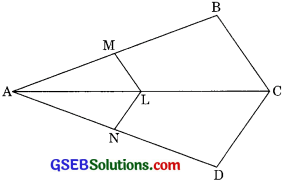Solution:
In ∆ABE
DF || AE
$$\frac{AD}{BD}$$ = $$\frac{FE}{BF}$$ ………(1) (by BPT)
In ∆ABC
DE || AC
$$\frac{AD}{DB}$$ = $$\frac{EC}{BE}$$ ……..(2) (ByBPT)
From equation (1) and (2)
$$\frac{FE}{BF}$$ = $$\frac{EC}{BE}$$
$$\frac{BF}{FE}$$ = $$\frac{BE}{EC}$$ (by Invertendo)Question 5.
In figure DE || OQ and DF || OR. Show that EF || QR. (Foreign 2008)
Solution:
In ∆POQ
DE || OQ
$$\frac{PE}{EQ}$$ = $$\frac{PD}{DO}$$ ………(1) (ByBPT)Now, in ∆PRO
DF || OR
Therefore = $$\frac{PD}{DO}$$ = $$\frac{PF}{FR}$$ ……..(2) (by BPT)
From eqn (1) and (2) we get
$$\frac{PE}{EQ}$$ = $$\frac{PF}{FR}$$
Therefore EF || QR (by converse of BVT)

Question 6.
In figure, A, B and C are points on OP, OQ and OR respectively such that AB || PQ and AC || PR. Show that BC || QR. (CBSE 2007)
Solution:
In ∆OPQ
AB || PQ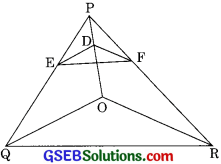then = $$\frac{OA}{AP}$$ = $$\frac{OB}{BQ}$$ ……(1) (by BPT)
In ∆OPR
AC || PR (given)
then = $$\frac{OA}{AP}$$ = $$\frac{OC}{CR}$$ ……. (2) (by BPT)
From equation (1) and (2)
$$\frac{OB}{BQ}$$ = $$\frac{OC}{CR}$$
Therefore BC || QR (by converse of BPT)Question 7.
Using theorem 6.1 (i.e. Basic proportionality theorem), prove that line drawn through the mid point of one side of a triangle parallel to another side bisects the third side (Recall that you have proved it in class IX) (CBSE 2012)
Solution:
Given:
∆ABC in which, D is the midpoint of AB and
DE || BC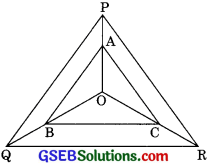To prove: E is the midpoint of AC
Proof: In ∆ABC
DE || BC (given)
then $$\frac{AD}{DB}$$ = $$\frac{AE}{EC}$$ ………(1) (by BPT)
∵ D is the midpoint of AB then
Putting this value in eqn (1) we get
$$\frac{DB}{DB}$$ = $$\frac{AE}{EC}$$
⇒ 1 = $$\frac{AE}{EC}$$
⇒ AE = EC
Hence E is the midpoint of AC.Question 8.
Using theorem 6.2 (i.e. Converse of basic proportionality theorem), prove that the line joining the midpoints of any two sides a triangle is a parallel to the third side (Recall that you have done it in class IX)
Solution:
Given: ∆EC in which D and E are the midpoints of sides AB and AC respectively. DE is the line joining D and E.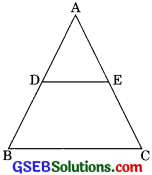To prove: DE || BC
Proof: D is the mid point of AB
∴ $$\frac{AD}{DB}$$ = 1 …….(1)
Similarly
$$\frac{AE}{EC}$$ = 1 (AE = EC)
From equation (1) and (2)
$$\frac{AD}{DB}$$ = $$\frac{AE}{EC}$$
Therefore DE || BC (by converse of BPT)

Question 9.
ABCD is a trapezium in which AB || DC and its diagonal intersect each other at the point O. Show that = $$\frac{AO}+{BO}$$ = $$\frac{CO}{DO}$$ (CBSE 2004)
Solution:
Given: ABCD is a trapezium in which AB || DC and diagonals of trapezium intersect each other at O.
To prove: = $$\frac{AO}{BO}$$ = $$\frac{CO}{DO}$$
Construction: Through O, draw a line 0E parallel to AB which intersect AD at E.
OE || DC
AB || DC and OE || AB then OE ||DC
then = $$\frac{AO}{CO}$$ = $$\frac{AE}{DE}$$ ……..(1) (By BPT)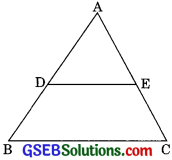In ∆ DBA
OE || AB
$$\frac{DE}{AE}$$ = $$\frac{DO}{BO}$$ (By BPT)
⇒ $$\frac{AE}{DE}$$ = $$\frac{BO}{DO}$$ (by Invertendo) ……(2)
From equations (1) and (2)
$$\frac{AO}{CO}$$ = $$\frac{BO}{DO}$$
⇒ $$\frac{AO}{BO}$$ = $$\frac{CO}{DO}$$ (by Alternendo)Question 10.
The diagonals of quadrilateral ABCD intersect each other at the point O such that $$\frac{AO}{BO}$$ = $$\frac{CO}{DO}$$ , show that ABCD is a trapezium. (CBSE 2012)
Solution:
Given: ABCD is a quadrilateral whose diagonals intersect each other at O such that
$$\frac{AO}+{BO}$$ = $$\frac{CO}+{DO}$$
To Prove: ABCD is a trapezium.
Construction: Draw a line 0E AB which intersect AD at E.
Proof: In ∆DAB
DO || DE (by construction)
then = $$\frac{DO}{BO}$$ = $$\frac{DE}{AE}$$ ……….(1)
But = $$\frac{AO}{BO}$$ = $$\frac{CO}{DO}$$ (Given)
⇒$$\frac{DO}{BO}$$ = $$\frac{CO}{AO}$$ ……..(2)from equation (1) and (2)
$$\frac{CO}{AO}$$ = $$\frac{DE}{AE}$$
or $$\frac{AO}{CO}$$ = $$\frac{AE}{DE}$$ (by Invertendo)